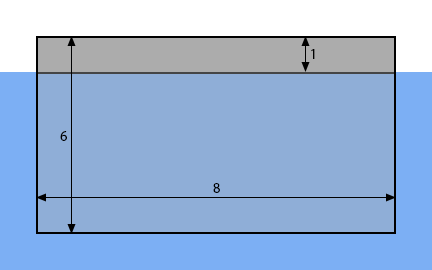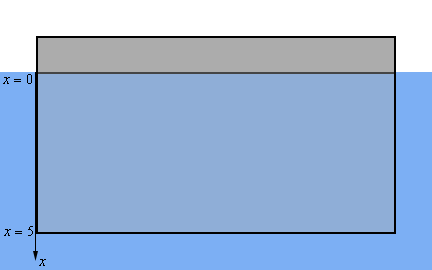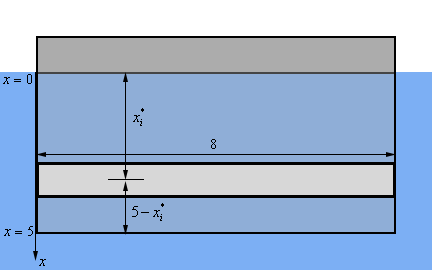Paul's Online Notes
Home / Calculus II / Applications of Integrals / Hydrostatic Pressure and Force
Show Mobile Notice Show All Notes Hide All Notes
Mobile Notice
You appear to be on a device with a "narrow" screen width (i.e. you are probably on a mobile phone). Due to the nature of the mathematics on this site it is best views in landscape mode. If your device is not in landscape mode many of the equations will run off the side of your device (should be able to scroll to see them) and some of the menu items will be cut off due to the narrow screen width.

### Section 8.4 : Hydrostatic Pressure and Force

1. Find the hydrostatic force on the plate submerged in water as shown in the image below.

Consider the top of the blue “box” to be the surface of the water in which the plate is submerged. Note as well that the dimensions in the image will not be perfectly to scale in order to better fit the plate in the image. The lengths given in the image are in meters.Show All Steps Hide All Steps

Hint : Start off by defining an “axis system” for the figure.
Start Solution

The first thing we should do is define an axis system for the portion of the plate that is below the water.Note that we started the $$x$$-axis at the surface of the water and by doing this $$x$$ will give the depth of any point on the plate below the surface of the water. This in turn means that the bottom of the plate will be defined by $$x = 5$$.

It is always useful to define some kind of axis system for the plate to help with the rest of the problem. There are lots of ways to actually define the axis system and how we define them will in turn affect how we work the rest of the problem. There is nothing special about one definition over another but there is often an “easier” axis definition and by “easier’ we mean is liable to make some portions of the rest of the work go a little easier.

Hint : At this point it would probably be useful to break up the plate into horizontal strips and get a sketch of a representative strip.
Show Step 2

As we did in the notes we’ll break up the portion of the plate that is below the surface of the water into $$n$$ horizontal strips of width $$\Delta \,x$$ and we’ll let each strip be defined by the interval $$\left[ {{x_{i - 1}},\,\,{x_i}} \right]$$ with $$i = 1,2,3, \ldots n$$. Finally, we’ll let $$x_i^*$$ be any point that is in the interval and hence will be some point on the strip.

Below is yet another sketch of the plate only this time we’ve got a representative strip sketched on the plate. Note that the strip is “thicker” than the strip really should be but it will make it easier to see what the strip looks like and get all of the appropriate lengths clearly listed.Now $$x_i^*$$ is a point from the interval defining the strip and so, for sufficiently thin strips, it is safe to assume that the strip will be at the point $$x_i^*$$ below the surface of the water as shown in the figure above. In other words, the strip is a distance of $$x_i^*$$ below the surface of the water.

Also, because our plate is a rectangle we know that each strip will have a width of 8.

Hint : What is the hydrostatic pressure and force on the representative strip?
Show Step 3

We’ll assume that the strip is sufficiently thin so the hydrostatic pressure on the strip will be constant and is given by,

${P_i} = \rho g{d_i} = \left( {1000} \right)\left( {9.81} \right)x_i^* = 9810x_i^*$

This, in turn, means that the hydrostatic force on each strip is given by,

${F_i} = {P_i}{A_i} = \left( {9810x_i^*} \right)\left[ {\left( 8 \right)\left( {\Delta \,x} \right)} \right] = 78480x_i^*\Delta \,x$
Hint : How can we use the result from the previous step to approximate the total hydrostatic force on the plate and how can we modify that to get an expression for the actual hydrostatic force on the plate?
Show Step 4

We can now approximate the total hydrostatic force on plate as the sum off the force on each of the strips. Or,

$F \approx \sum\limits_{i = 1}^n {78480x_i^*\Delta \,x}$

Now, we can get an expression for the actual hydrostatic force on the plate simply by letting $$n$$ go to infinity.

Or in other words, we take the limit as follows,

$F = \mathop {\lim }\limits_{n \to \infty } \sum\limits_{i = 1}^n {78480x_i^*\Delta \,x}$
Hint : You do recall the definition of the definite integral don’t you?
Show Step 5

Finally, we know from the definition of the definite integral that this is nothing more than the following definite integral that we can easily compute.

$F = \int_{0}^{5}{{78480x\,dx}} = \left. {39240{x^2}} \right|_0^5 = \require{bbox} \bbox[2pt,border:1px solid black]{{981,000N}}$